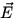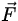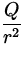# Electric Forces and Fields

The amount of attraction or repulsion between charged objects can be put in quantitative terms by the introduction of the electric force. The simplest case to consider is the force between two point charges (charges with a negligible size). Experiments by Coulomb and others uncovered the following formula for the forcebetween two such charges Q1 and Q2 separated by a distance r , as in Fig. 1.1:The magnitude of the force between the charges is

 F = k(2)

where k is a constant called Coulomb's constant:

 k = 9.0 x 109(3)

The direction of the force is along a line joining the two charges. It is repulsive if the charges have the same sign and is attractive if the charges have opposite sign.

If there are more than two charges present, then the force on any one charge must be found by adding vectorially the forces found by Coulomb's law (1.2) between each pair of charges.

It is convenient for many applications to introduce the concept of the electric field, conventionally denoted by. Suppose we have a background'' distribution of charge Q1,Q2,...,Qn in some region of space, and measure the forceon a charge q placed nearby. The electric fieldassociated with this charge distribution is defined through the relation= q. (4)

The units ofare thus seen to be N/C. In a sense, the charge q is a test charge which probes the strength at different points of the potential electric force due to the charges Q1,Q2,...,Qn . Note that, for a given electric field, the force on a positive charge is opposite in direction to the force on a negative charge.

For a single point charge Q the electric field a distance r away is found from Eqs.(1.2,1.4) to have the magnitude

 E = k, (5)

with a direction equal to the direction of the force on a positive test charge placed at the point of interest. As with the electric force, the electric field due to multiple point charges must be found by adding vectorially the electric field found by Eq.(1.5) for each individual charge.Next: Electric work and potential Up: Electric Fields and Potentials Previous: Electric Charge